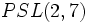# Quasisimple group

This article defines a group property: a property that can be evaluated to true/false for any given group, invariant under isomorphism
View a complete list of group properties
VIEW RELATED: Group property implications | Group property non-implications |Group metaproperty satisfactions | Group metaproperty dissatisfactions | Group property satisfactions | Group property dissatisfactions
This is a variation of simplicity|Find other variations of simplicity | Read a survey article on varying simplicity

VIEW RELATED: Analogues of this | Variations of this | Opposites of this |
View a complete list of semi-basic definitions on this wiki

## Definition

### Symbol-free definition

A group is said to be quasisimple if it is perfect and its inner automorphism group is simple.

### Definition with symbols

A group$G$ is said to be quasisimple if both the following hold:

•$G$ is perfect, that is,$G' = G$
• The inner automorphism group of$G$ is a simple group, that is,$G/Z(G)$ is simple (where$Z(G)$ denotes the center of$G$).

## Classification

Further information: Classification of finite simple groups

The finite quasisimple groups can be completely classified in terms of the finite simple non-abelian groups. Specifically, for each finite simple non-abelian group$S$, consider the Schur multiplier and the corresponding Schur covering group (the unique universal central extension)$\hat S$ with its map to$G$. The quasisimple groups with inner automorphism group$S$ are precisey the groups$S$ such that the map$\hat S \to S$ can be factored in terms of a surjective map$\hat S \to G$ and a surjective map$G \to S$.

In particular, by the fourth isomorphism theorem, these correspond precisely to the quotient groups (and hence to the subgroups, because of abelianness) of$\hat S/S$, which is the Schur multiplier of$S$. In particular, for each finite simple non-abelian groups, there are finitely many quasisimple groups associated with it.

## Examples

Finite simple non-abelian group Order Schur multiplier Schur covering group Quasisimple groups with this simple group as the inner automorphism group
alternating group:A5 60 cyclic group:Z2 special linear group:SL(2,5) alternating group:A5, special linear group:SL(2,5)
projective special linear group:PSL(3,2) (isomorphic to$PSL(2,7)$) 168 cyclic group:Z2 special linear group:SL(2,7) projective special linear group:PSL(3,2), special linear group:SL(2,7)
alternating group:A6 360 cyclic group:Z6 Schur cover of alternating group:A6 alternating group:A6, special linear group:SL(2,9), triple cover of alternating group:A6, Schur cover of alternating group:A6
projective special linear group:PSL(2,8) 504 trivial group projective special linear group:PSL(2,8) projective special linear group:PSL(2,8)
projective special linear group:PSL(2,11) 660 cyclic group:Z2 special linear group:SL(2,11) projective special linear group:PSL(2,11), special linear group:SL(2,11)